• Размер: 992.5 Кб
• Количество слайдов: 26

## Описание презентации Slides by John F. Hall Animations by Anthony по слайдам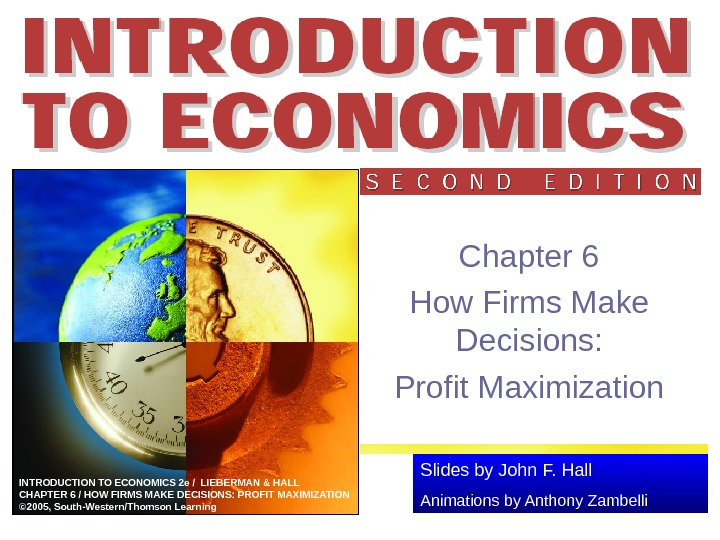Slides by John F. Hall Animations by Anthony Zambelli. INTRODUCTION TO ECONOMICS 2 e / LIEBERMAN & HALL CHAPTER 6 / HOW FIRMS MAKE DECISIONS: PROFIT MAXIMIZATION © 2005, South-Western/Thomson Learning Chapter 6 How Firms Make Decisions: Profit Maximization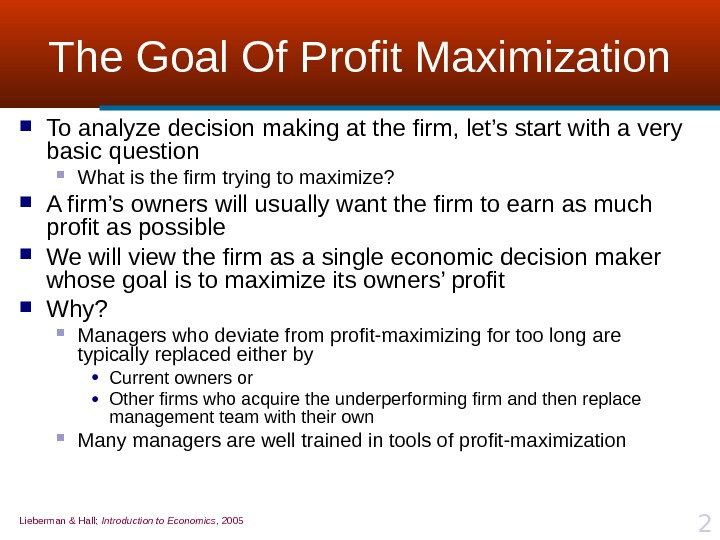Lieberman & Hall; Introduction to Economics , 2005 2 The Goal Of Profit Maximization To analyze decision making at the firm, let’s start with a very basic question What is the firm trying to maximize? A firm’s owners will usually want the firm to earn as much profit as possible We will view the firm as a single economic decision maker whose goal is to maximize its owners’ profit Why? Managers who deviate from profit-maximizing for too long are typically replaced either by • Current owners or • Other firms who acquire the underperforming firm and then replace management team with their own Many managers are well trained in tools of profit-maximization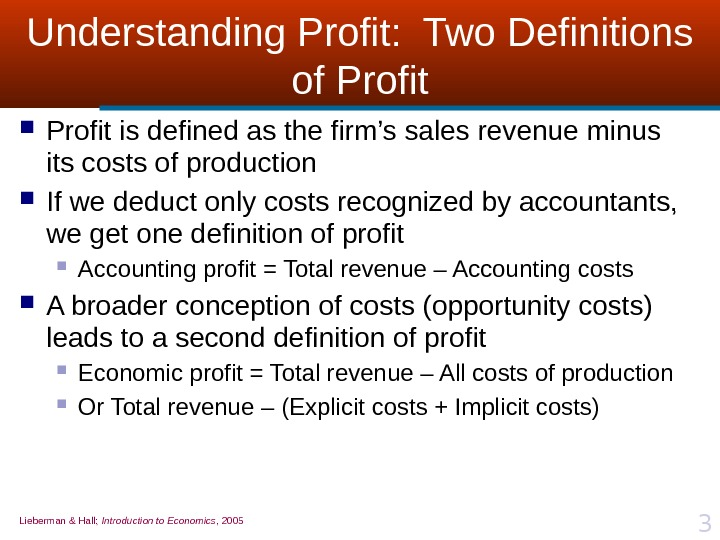Lieberman & Hall; Introduction to Economics , 2005 3 Understanding Profit: Two Definitions of Profit is defined as the firm’s sales revenue minus its costs of production If we deduct only costs recognized by accountants, we get one definition of profit Accounting profit = Total revenue – Accounting costs A broader conception of costs (opportunity costs) leads to a second definition of profit Economic profit = Total revenue – All costs of production Or Total revenue – (Explicit costs + Implicit costs)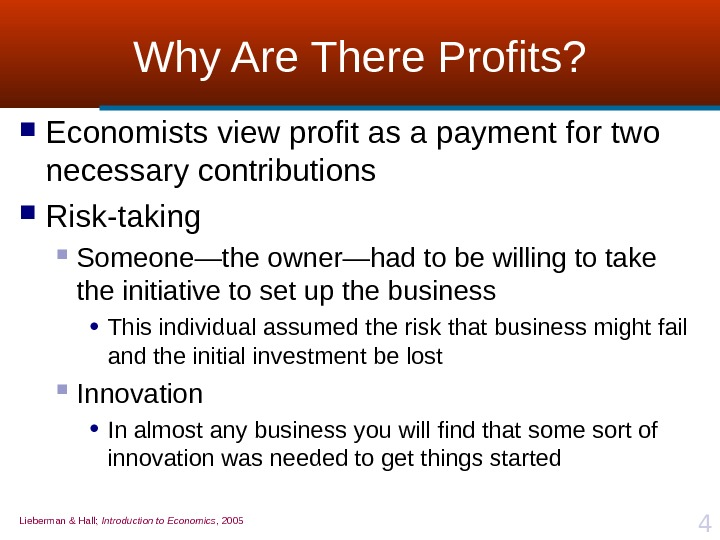Lieberman & Hall; Introduction to Economics , 2005 4 Why Are There Profits? Economists view profit as a payment for two necessary contributions Risk-taking Someone—the owner—had to be willing to take the initiative to set up the business • This individual assumed the risk that business might fail and the initial investment be lost Innovation • In almost any business you will find that some sort of innovation was needed to get things started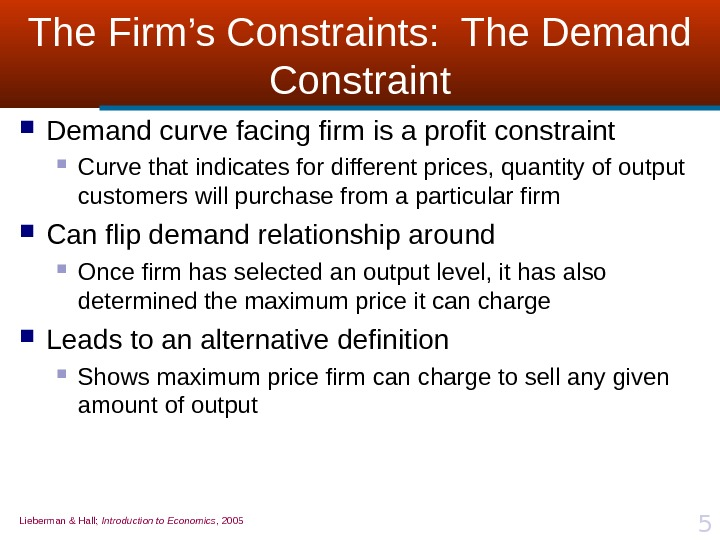Lieberman & Hall; Introduction to Economics , 2005 5 The Firm’s Constraints: The Demand Constraint Demand curve facing firm is a profit constraint Curve that indicates for different prices, quantity of output customers will purchase from a particular firm Can flip demand relationship around Once firm has selected an output level, it has also determined the maximum price it can charge Leads to an alternative definition Shows maximum price firm can charge to sell any given amount of output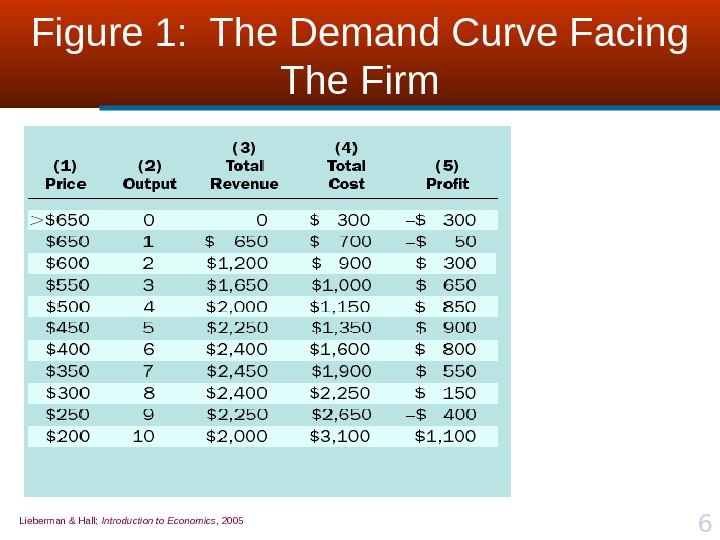Lieberman & Hall; Introduction to Economics , 2005 6 Figure 1: The Demand Curve Facing The Firm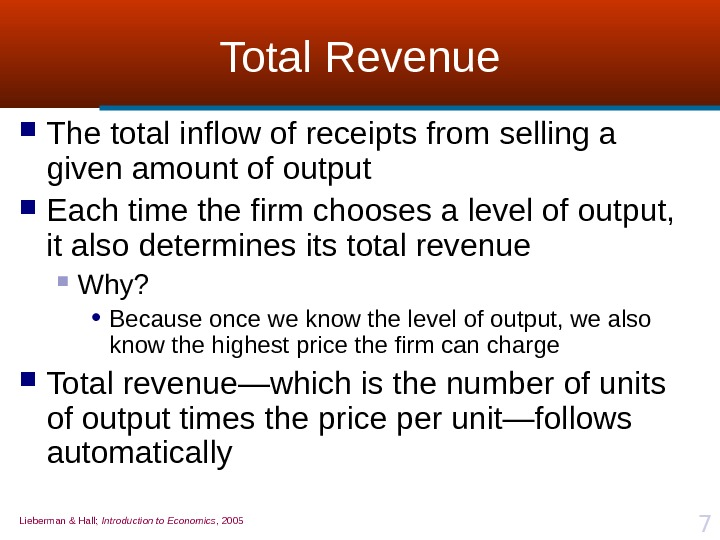Lieberman & Hall; Introduction to Economics , 2005 7 Total Revenue The total inflow of receipts from selling a given amount of output Each time the firm chooses a level of output, it also determines its total revenue Why? • Because once we know the level of output, we also know the highest price the firm can charge Total revenue—which is the number of units of output times the price per unit—follows automatically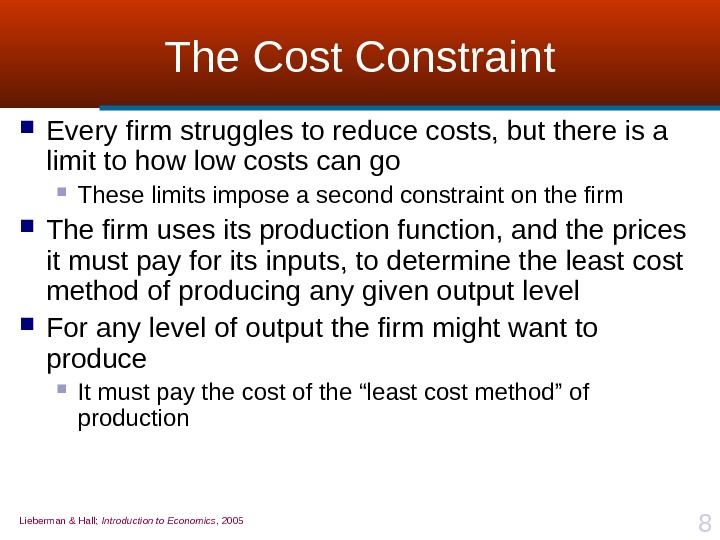Lieberman & Hall; Introduction to Economics , 2005 8 The Cost Constraint Every firm struggles to reduce costs, but there is a limit to how low costs can go These limits impose a second constraint on the firm The firm uses its production function, and the prices it must pay for its inputs, to determine the least cost method of producing any given output level For any level of output the firm might want to produce It must pay the cost of the “least cost method” of production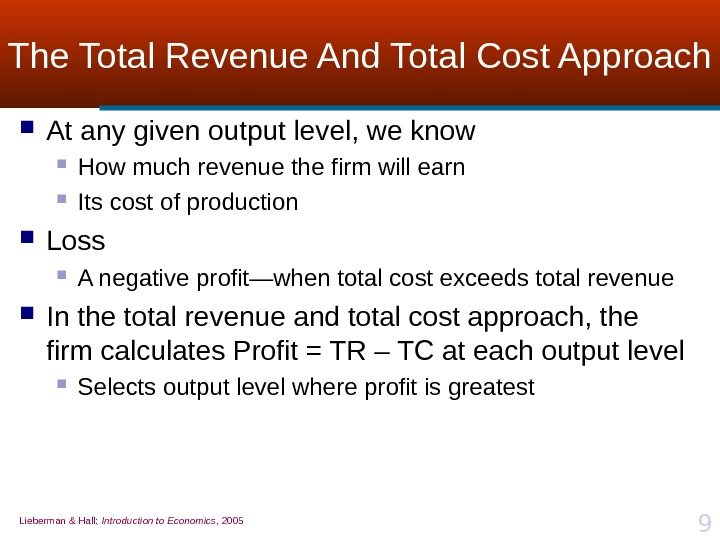Lieberman & Hall; Introduction to Economics , 2005 9 The Total Revenue And Total Cost Approach At any given output level, we know How much revenue the firm will earn Its cost of production Loss A negative profit—when total cost exceeds total revenue In the total revenue and total cost approach, the firm calculates Profit = TR – TC at each output level Selects output level where profit is greatest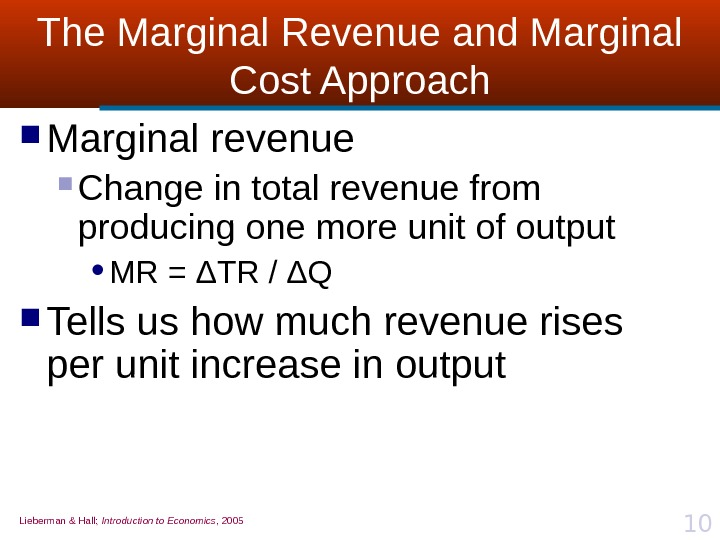Lieberman & Hall; Introduction to Economics , 2005 10 The Marginal Revenue and Marginal Cost Approach Marginal revenue Change in total revenue from producing one more unit of output • MR = Δ TR / Δ Q Tells us how much revenue rises per unit increase in output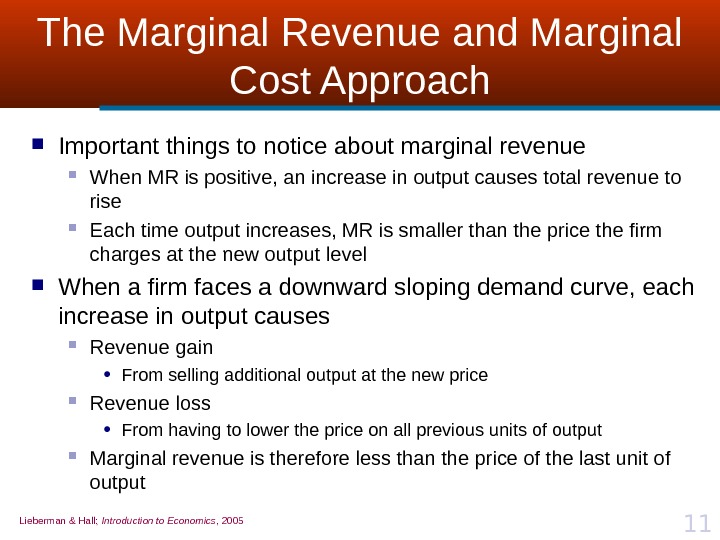Lieberman & Hall; Introduction to Economics , 2005 11 The Marginal Revenue and Marginal Cost Approach Important things to notice about marginal revenue When MR is positive, an increase in output causes total revenue to rise Each time output increases, MR is smaller than the price the firm charges at the new output level When a firm faces a downward sloping demand curve, each increase in output causes Revenue gain • From selling additional output at the new price Revenue loss • From having to lower the price on all previous units of output Marginal revenue is therefore less than the price of the last unit of output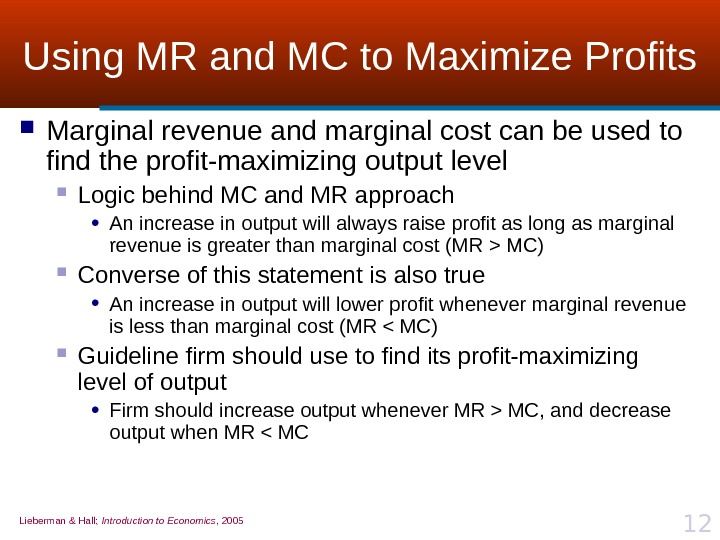Lieberman & Hall; Introduction to Economics , 2005 12 Using MR and MC to Maximize Profits Marginal revenue and marginal cost can be used to find the profit-maximizing output level Logic behind MC and MR approach • An increase in output will always raise profit as long as marginal revenue is greater than marginal cost (MR > MC) Converse of this statement is also true • An increase in output will lower profit whenever marginal revenue is less than marginal cost (MR MC, and decrease output when MR < M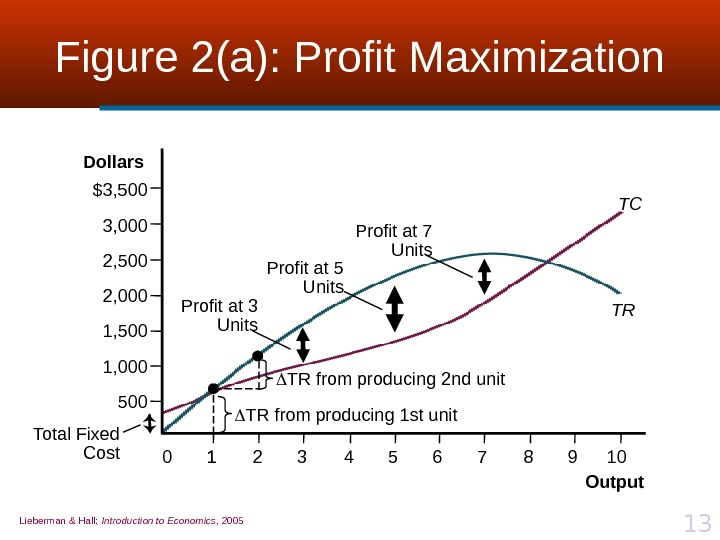Lieberman & Hall; Introduction to Economics , 2005 13 Figure 2(a): Profit Maximization Total Fixed Cost TC TR TR from producing 2 nd unit TR from producing 1 st unit. Profit at 3 Units Profit at 5 Units\$3, 500 3, 000 2, 500 2, 000 1, 500 1, 000 500 Output. Dollars 1 210 3 4 5 6 7 8 9 10 Profit at 7 Units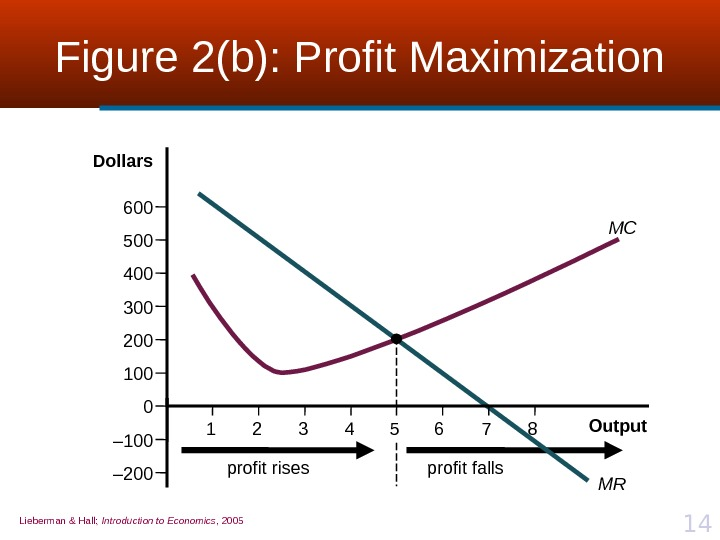Lieberman & Hall; Introduction to Economics , 2005 14 Figure 2(b): Profit Maximization profit rises profit falls MC MR 0600 500 400 300 200 100 – 200 Output. Dollars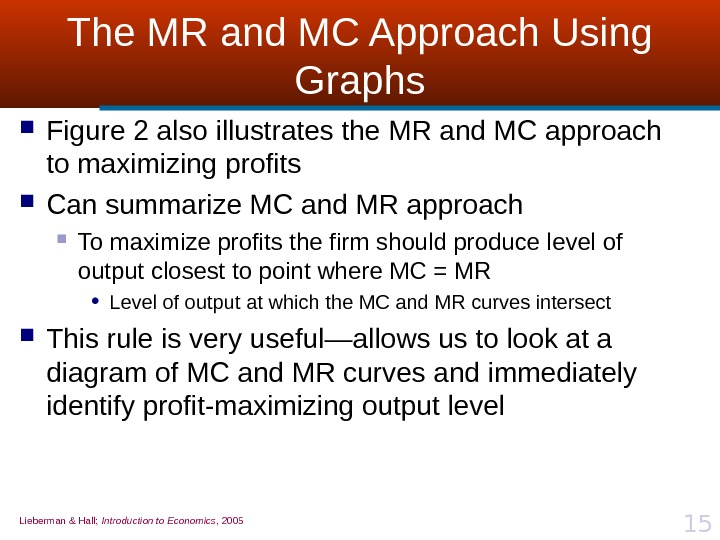Lieberman & Hall; Introduction to Economics , 2005 15 The MR and MC Approach Using Graphs Figure 2 also illustrates the MR and MC approach to maximizing profits Can summarize MC and MR approach To maximize profits the firm should produce level of output closest to point where MC = MR • Level of output at which the MC and MR curves intersect This rule is very useful — allows us to look at a diagram of MC and MR curves and immediately identify profit-maximizing output level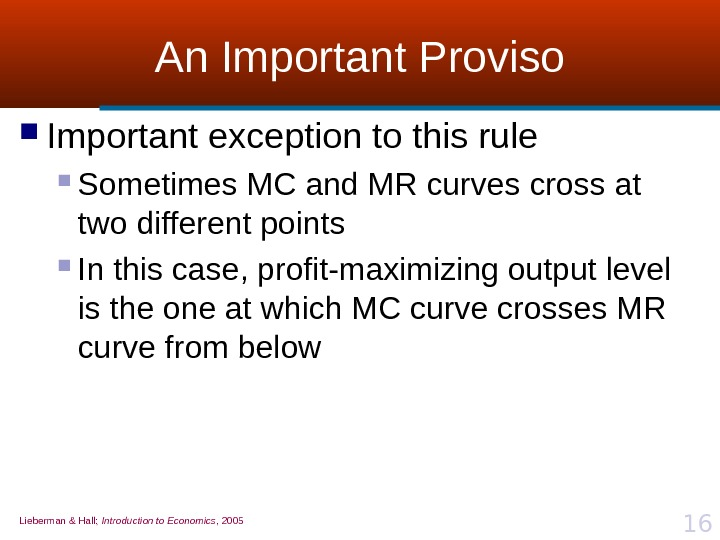Lieberman & Hall; Introduction to Economics , 2005 16 An Important Proviso Important exception to this rule Sometimes MC and MR curves cross at two different points In this case, profit-maximizing output level is the one at which MC curve crosses MR curve from below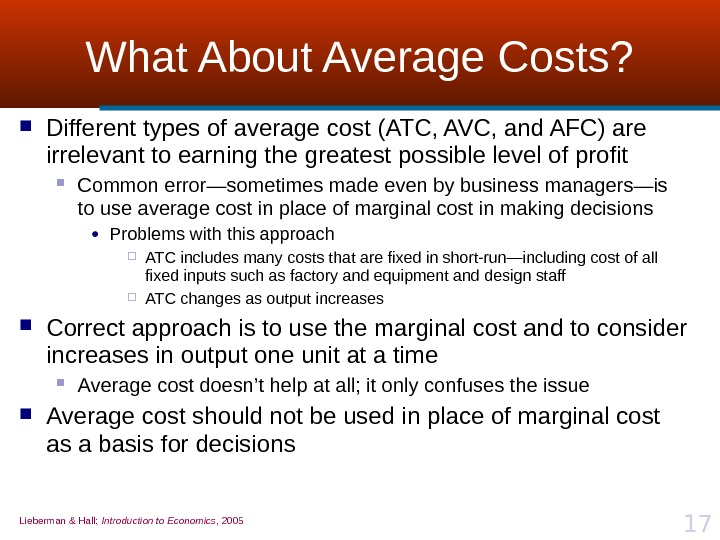Lieberman & Hall; Introduction to Economics , 2005 17 What About Average Costs? Different types of average cost (ATC, AVC, and AFC) are irrelevant to earning the greatest possible level of profit Common error—sometimes made even by business managers—is to use average cost in place of marginal cost in making decisions • Problems with this approach ATC includes many costs that are fixed in short-run—including cost of all fixed inputs such as factory and equipment and design staff ATC changes as output increases Correct approach is to use the marginal cost and to consider increases in output one unit at a time Average cost doesn’t help at all; it only confuses the issue Average cost should not be used in place of marginal cost as a basis for decisions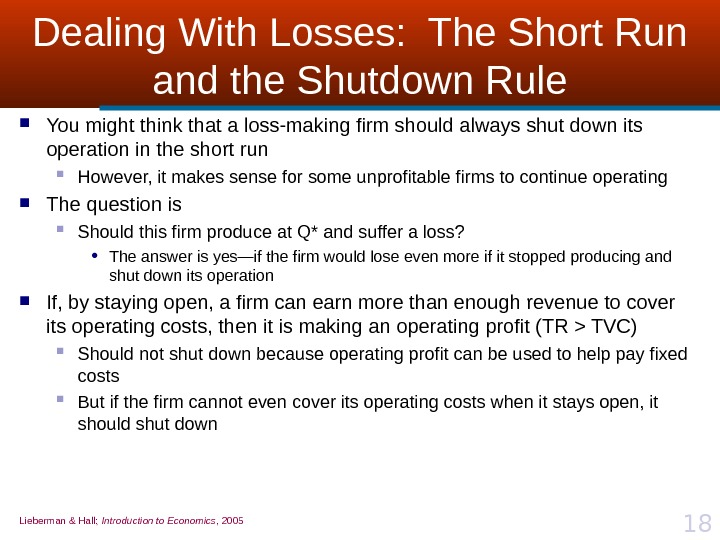Lieberman & Hall; Introduction to Economics , 2005 18 Dealing With Losses: The Short Run and the Shutdown Rule You might think that a loss-making firm should always shut down its operation in the short run However, it makes sense for some unprofitable firms to continue operating The question is Should this firm produce at Q * and suffer a loss? • The answer is yes—if the firm would lose even more if it stopped producing and shut down its operation If, by staying open, a firm can earn more than enough revenue to cover its operating costs, then it is making an operating profit (TR > TVC) Should not shut down because operating profit can be used to help pay fixed costs But if the firm cannot even cover its operating costs when it stays open, it should shut down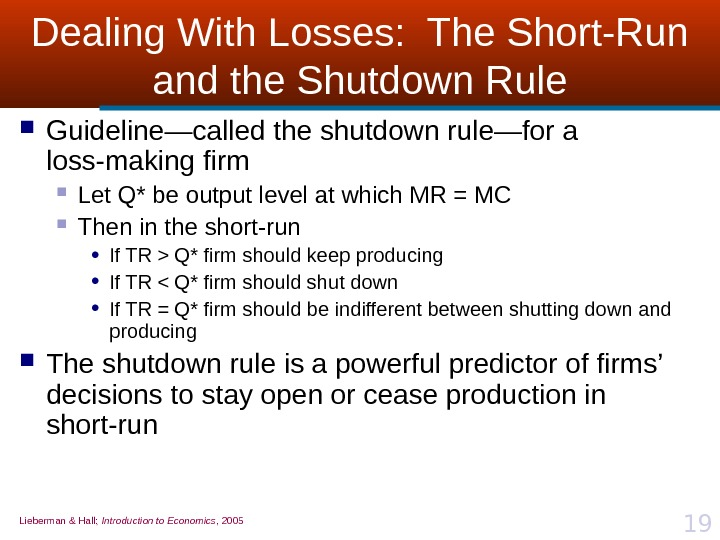Lieberman & Hall; Introduction to Economics , 2005 19 Dealing With Losses: The Short-Run and the Shutdown Rule Guideline—called the shutdown rule—for a loss-making firm Let Q* be output level at which MR = MC Then in the short-run • If TR > Q* firm should keep producing • If TR < Q* firm should shut down • If TR = Q* firm should be indifferent between shutting down and producing The shutdown rule is a powerful predictor of firms’ decisions to stay open or cease production in short-run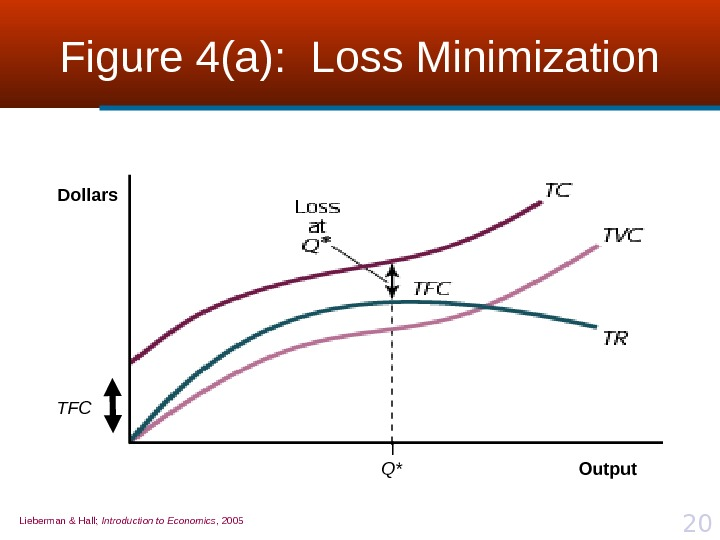Lieberman & Hall; Introduction to Economics , 2005 20 Figure 4(a): Loss Minimization Q*Dollars Output. T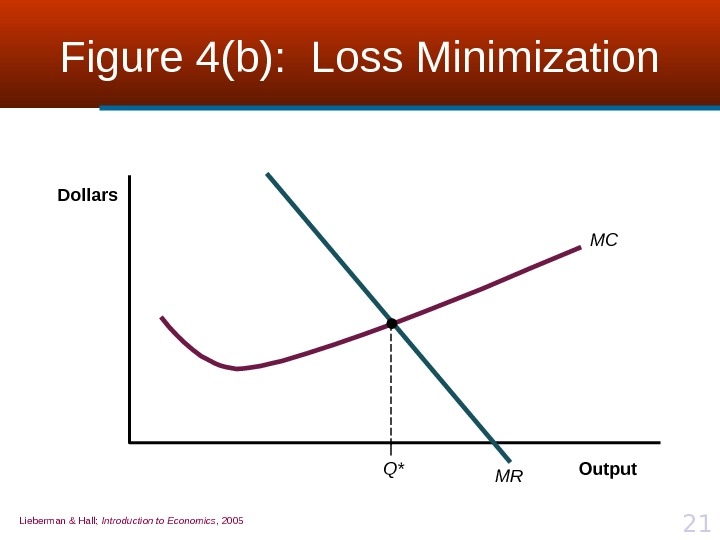Lieberman & Hall; Introduction to Economics , 2005 21 Figure 4(b): Loss Minimization MC MR Q*Dollars Output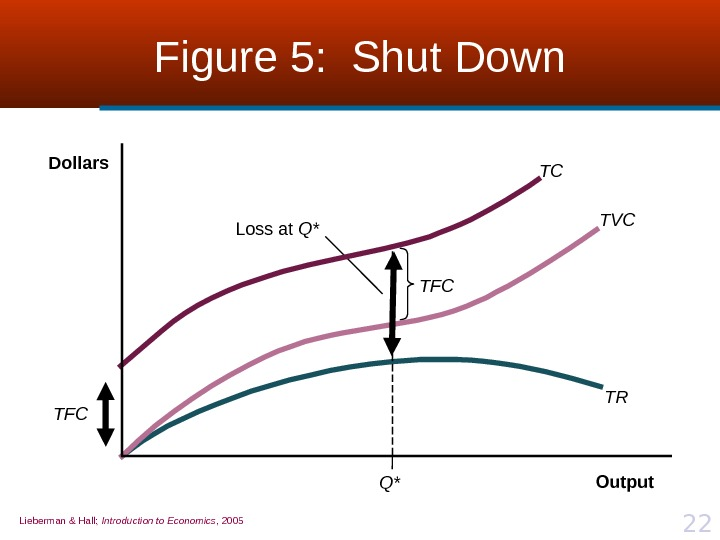Lieberman & Hall; Introduction to Economics , 2005 22 Figure 5: Shut Down Q* TC TRTVC TFC Loss at Q*Dollars Output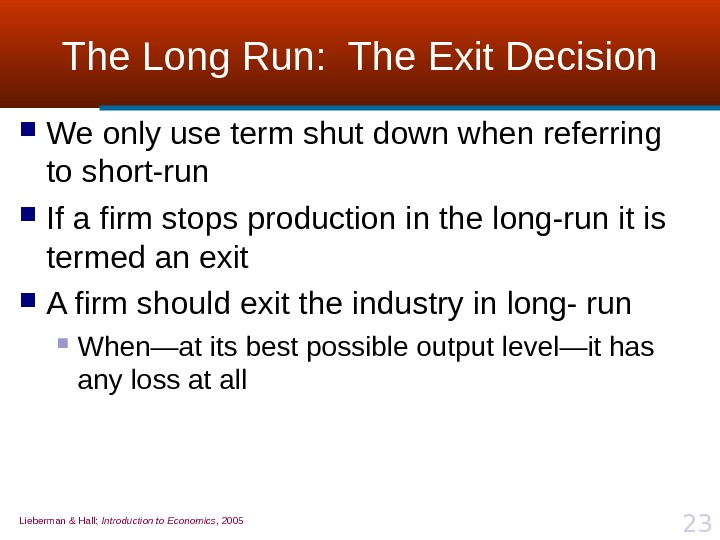Lieberman & Hall; Introduction to Economics , 2005 23 The Long Run: The Exit Decision We only use term shut down when referring to short-run If a firm stops production in the long-run it is termed an exit A firm should exit the industry in long- run When—at its best possible output level—it has any loss at all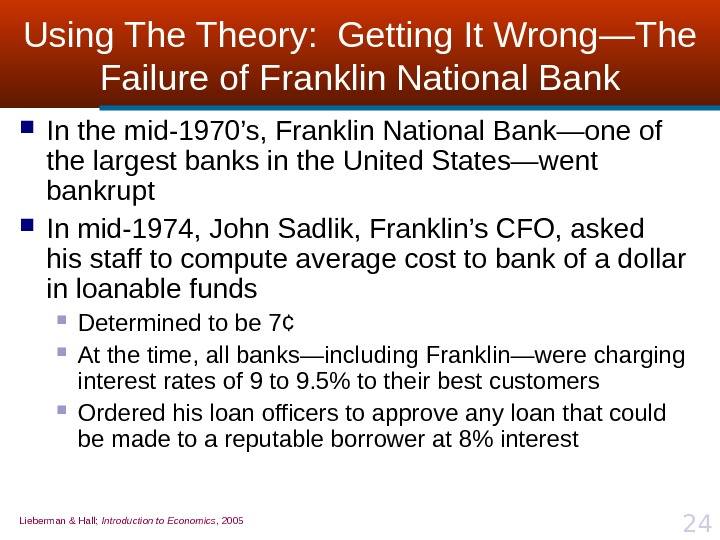Lieberman & Hall; Introduction to Economics , 2005 24 Using Theory: Getting It Wrong — The Failure of Franklin National Bank In the mid-1970’s, Franklin National Bank—one of the largest banks in the United States—went bankrupt In mid-1974, John Sadlik, Franklin’s CFO, asked his staff to compute average cost to bank of a dollar in loanable funds Determined to be 7 ¢ At the time, all banks—including Franklin—were charging interest rates of 9 to 9. 5% to their best customers Ordered his loan officers to approve any loan that could be made to a reputable borrower at 8% interest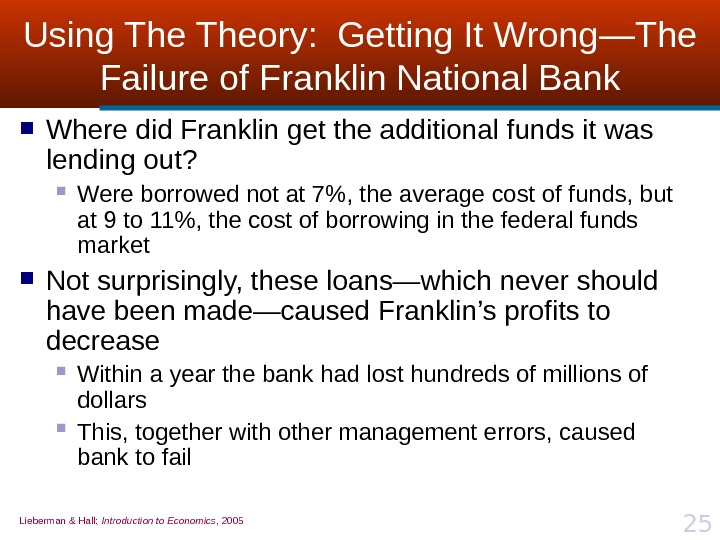Lieberman & Hall; Introduction to Economics , 2005 25 Using Theory: Getting It Wrong — The Failure of Franklin National Bank Where did Franklin get the additional funds it was lending out? Were borrowed not at 7%, the average cost of funds, but at 9 to 11%, the cost of borrowing in the federal funds market Not surprisingly, these loans—which never should have been made—caused Franklin’s profits to decrease Within a year the bank had lost hundreds of millions of dollars This, together with other management errors, caused bank to fail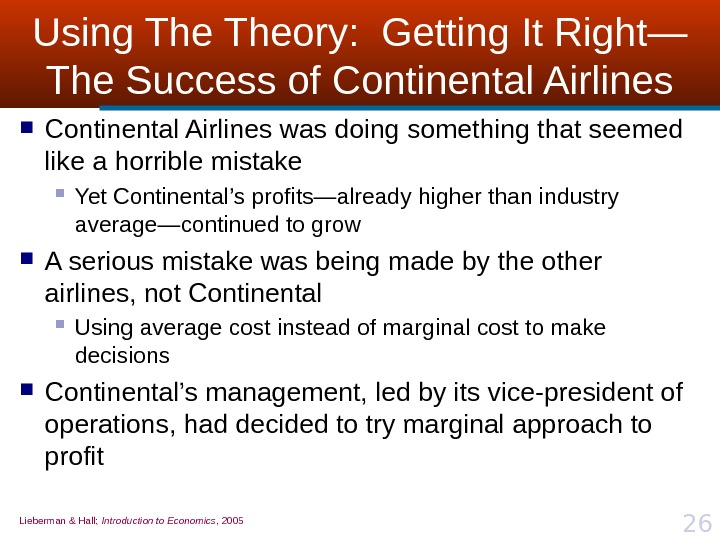Lieberman & Hall; Introduction to Economics , 2005 26 Using Theory: Getting It Right — The Success of Continental Airlines was doing something that seemed like a horrible mistake Yet Continental’s profits—already higher than industry average—continued to grow A serious mistake was being made by the other airlines, not Continental Using average cost instead of marginal cost to make decisions Continental’s management, led by its vice-president of operations, had decided to try marginal approach to profit